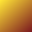#Swaping X and Y

It may take some ingenuity to exchange the values of two variables.

If we say X = Y and then Y = X we will find that the initial value of X has been lost after the first statement executes.

A common way to exchange two variables starts by saving one value in a temporary variable T = X. Then the exchange is completed with X = Y and then Y = T.

Some computer languages offer a swap operation that completes the exchange in with one statement X :=: Y.

Some computer languages offer a parallel assignment that has both assignments happening at once without interference [X, Y] = [Y, X].

Many sorting algorithms work by swapping carefully selected elements of a single array A[ j ] :=: A[ k ].